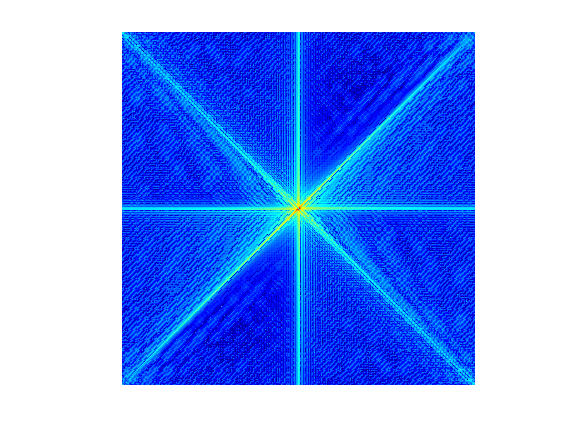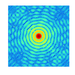# Pattern7Shanshan Wang on 22 Oct 2022
• 5
• 5
• 2
• 0
• 177
n = 2^10;
M = zeros(n);
I = 1:n;
x = I-n/2.5;
y = n/2.5-I;
[X,Y] = meshgrid(x,y);
R = 5;
A = (X.^2 - Y.^2 <= R^2);
M(A) = 1;
DP = fftshift(fft2(M));
imagesc(abs(log2(DP)))
colormap jet
axis image off##### Remix Tree
•••••